##### How to Use LOG() Function in PostgreSQL

PostgreSQL provides a built-in LOG() function to calculate the logarithm of a number to the base ten or the specified base. It is a mathematical function that accepts a numeric value, double precision value, or a numeric expression and retrieves the base 10 logarithms of the given number/expression.

This blog post will demonstrate the working of the LOG() function via practical examples. So, let’s get started.

How to Use LOG() Function in Postgres?

By default, the LOG() function calculates the base 10 logarithms of a number. However, you can specify the base of your choice as an argument to the LOG() function.

Syntax

Use one of the following syntaxes to calculate the logarithms of a number using the LOG() function:

`LOG(num);`

Or

`LOG(base, num);`

Here, "num" can be numeric or double precision value. Or it can be a numeric expression.

Example 1: Base 10 Logarithm

Let’s pass a number to the LOG() function to calculate the base 10 log of the input number:

`SELECT LOG(72);`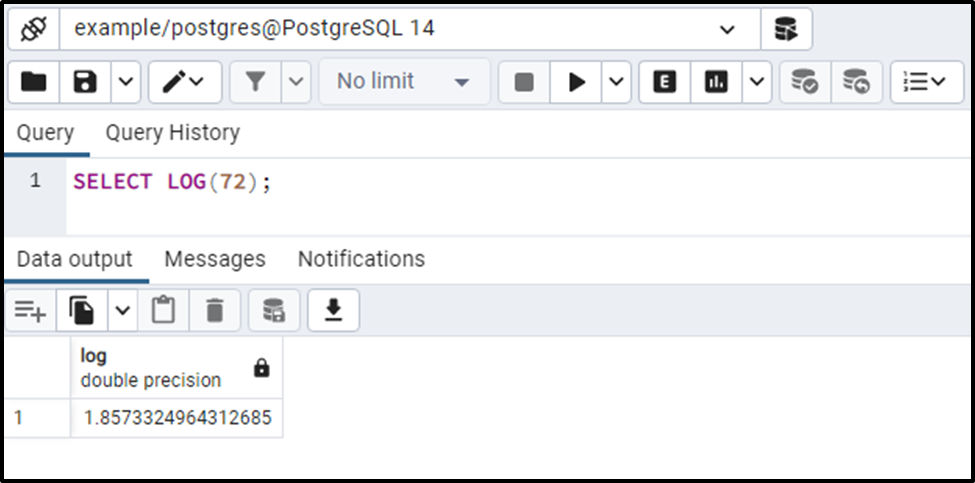The output shows that the LOG() function retrieves the base 10 logarithms of the “72”.

Example 2: Base 2 Logarithm

In this example, we will calculate the base 2 logarithm of a number:

`SELECT LOG(2.0, 72);`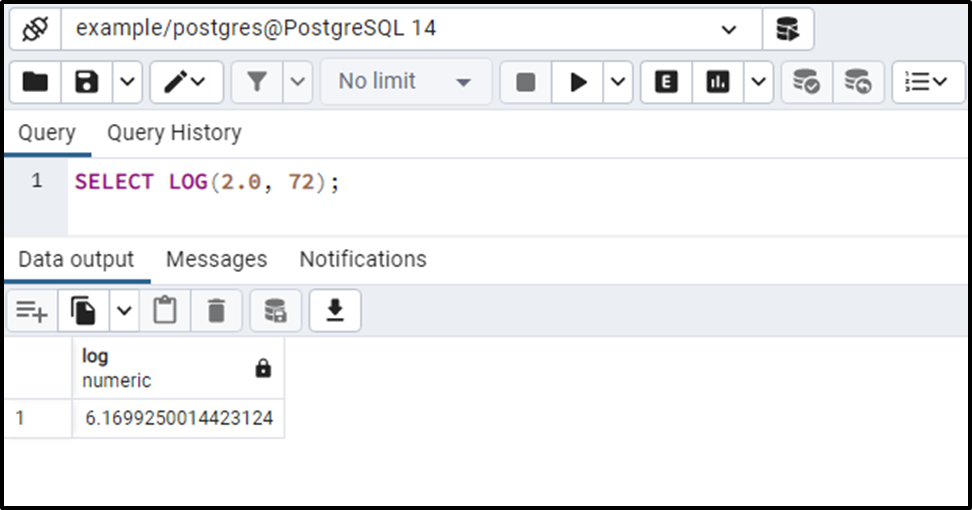The output shows that the LOG() function retrieves the base 2 logarithms of the input number.

Example 3: Pass an Expression to the LOG() Function

In this example, we will pass an expression to the LOG() function:

`SELECT LOG(10 * 2);`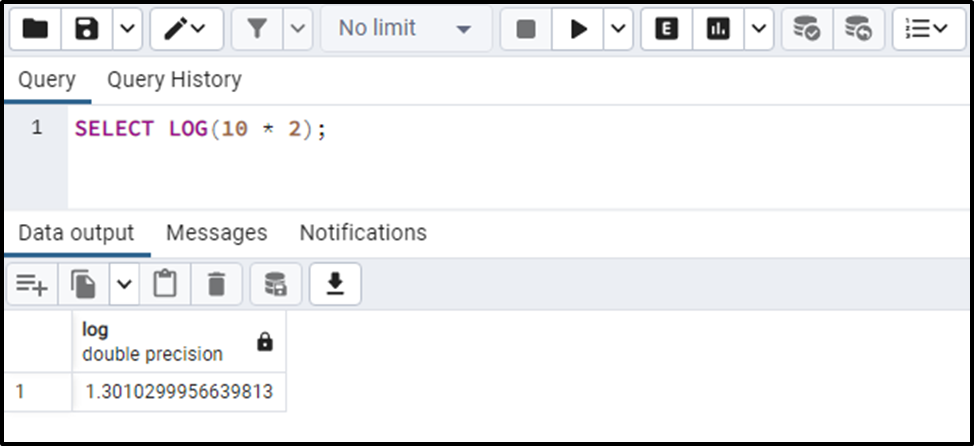The output authenticates the working of the LOG() function.

Example 4: How to Use the LOG() Function on Table’s Data?

Let’s create a sample table named exm_tab with two columns: exp_base and exp_num:

```CREATE TABLE exm_tab(
exp_base NUMERIC,
exp_num NUMERIC
);```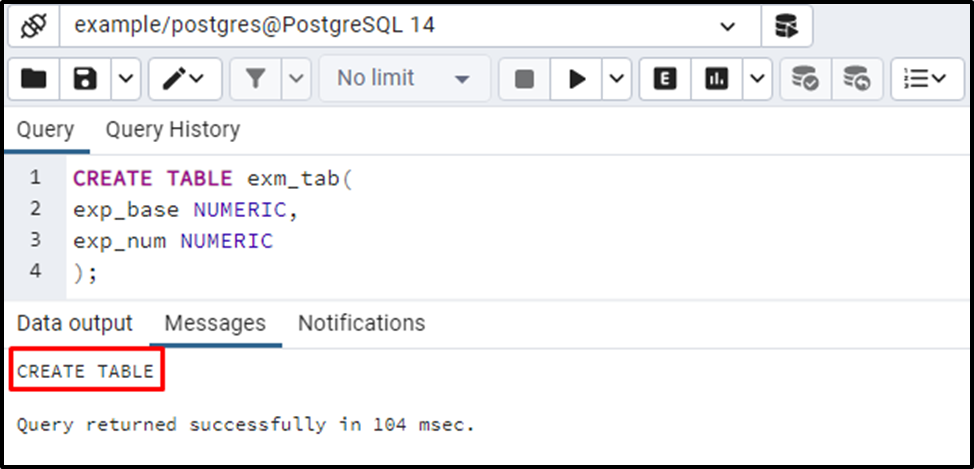Now we will insert some records into the newly created table:

```INSERT INTO exm_tab(exp_base, exp_num)
VALUES (10, 200.00),
(2, 200.00),
(10, 20),
(10, 20);```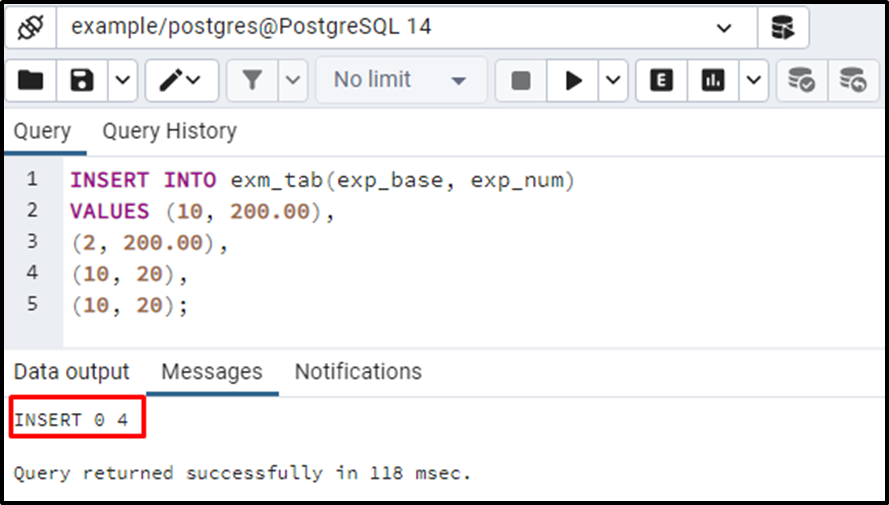To see the newly inserted data, execute the SELECT command as follows:

`SELECT * FROM exm_tab;`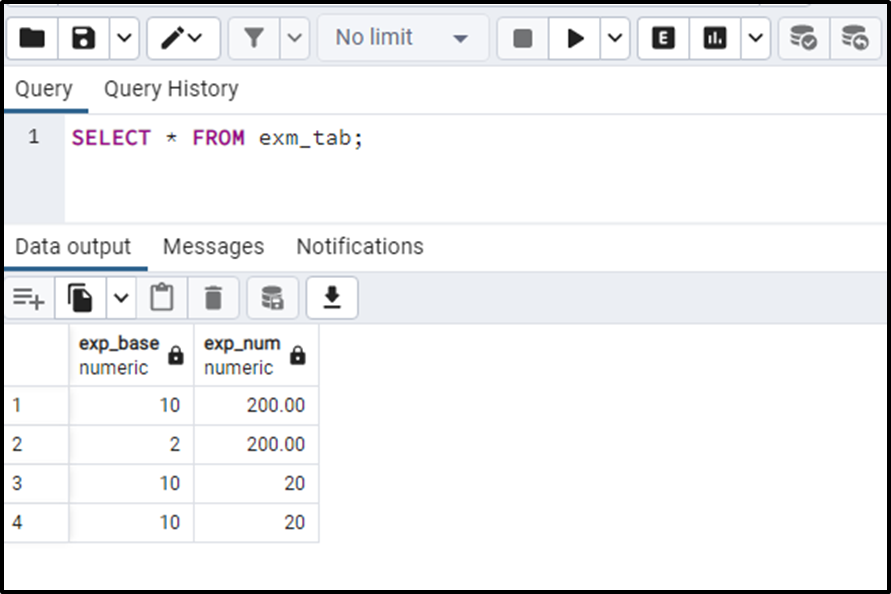Let’s utilize the LOG() function on the “exm_tab” table to calculate the logarithm of various numbers:

```SELECT LOG(exp_base, exp_num)
FROM exm_tab;```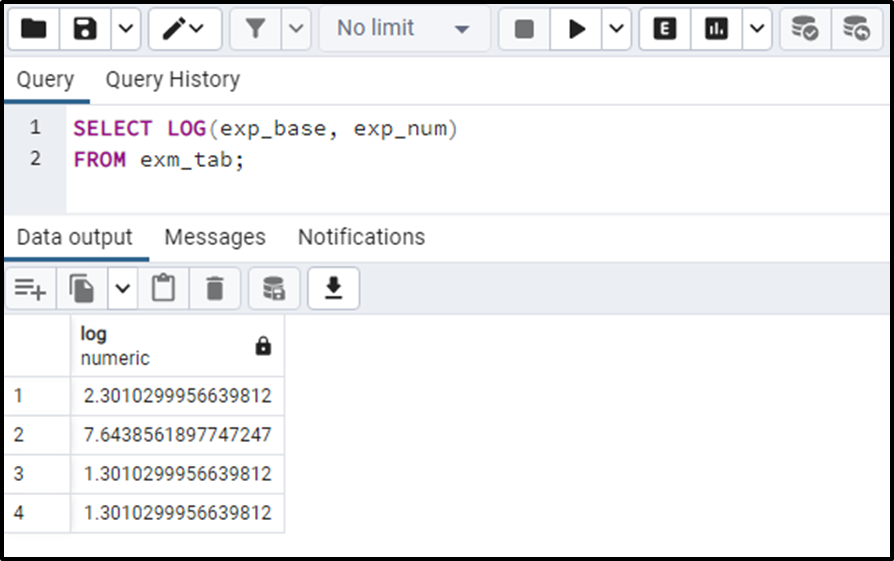This is how you can utilize the LOG() function on the table’s data.

Conclusion

PostgreSQL provides a built-in mathematical function named LOG() that calculates the logarithm of a number to the base ten or the specified base. By default, the LOG() function calculates the base 10 logarithms of a number. However, you can specify the base of your choice as an argument to the LOG() function. This blog post considered numerous use cases of the LOG() function and practical examples.